# Extract Day from Date – Excel & Google Sheets

This tutorial will demonstrate how to extract the day number from a date in Excel and Google Sheets.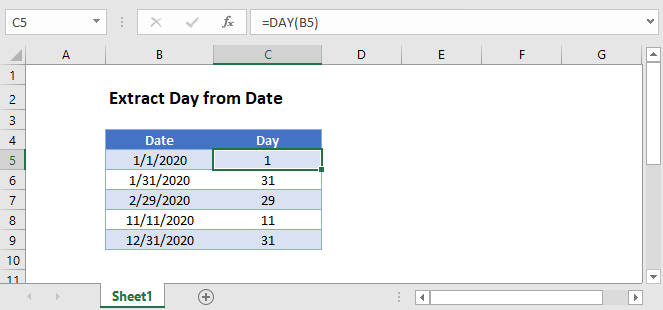## Extract Day from Date

### DAY Function

Extracting the day part of a date is really straightforward using the DAY Function:

``=DAY(B3)``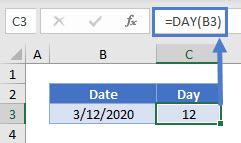The DAY Function returns the day from a date.

### Day From Date Stored as Text

If your Date is stored as text, first you must convert it from text to a valid date:

``=DATEVALUE(B3)``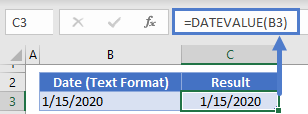## Date Format: Show only Day

Another way to display only the day part of a date is simply changing the cell to a custom date format. Select the date, then go to Format Cells > Custom, and enter one of these formats: dddd, ddd, dd, d.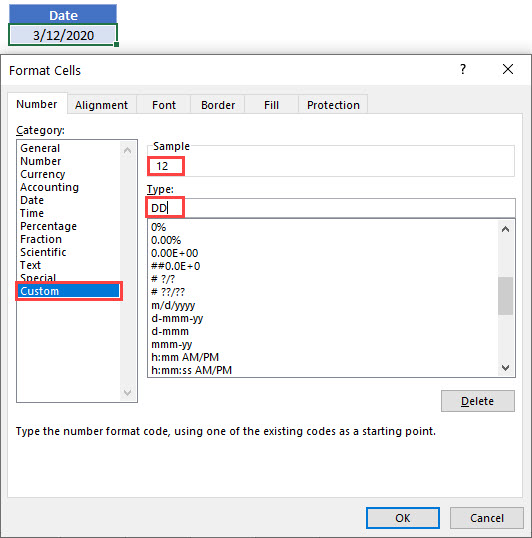In this example, the cell value is still the date March 12, 2020, but it is displayed as the number 12. Any formulas referring to B3 will recognize it as a date and not the number 12.

### TEXT Function

Another option is the TEXT Function with the format “dd”.

``=TEXT(B3,"dd")``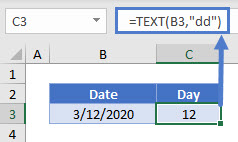In this example, we’re displaying the number 12 as text.

## Extract Day from Date in Google Sheets

These formulas work exactly the same in Google Sheets as in Excel.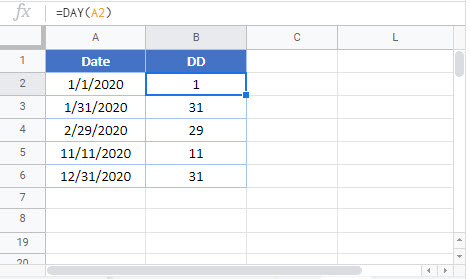### Excel Practice Worksheet

Practice Excel functions and formulas with our 100% free practice worksheets!